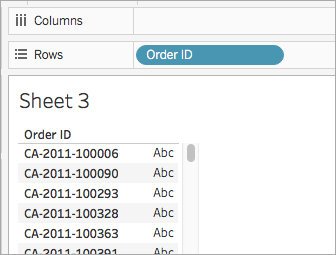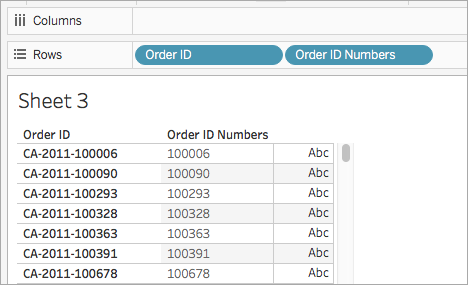# String Functions

This article introduces string functions and their uses in Tableau. It also demonstrates how to create a string calculation using an example.

## Why use string functions

String functions allow you to manipulate string data (i.e. data made of text). Tableau uses the current International Components for Unicode (ICU) library when comparing strings. The way strings are sorted and compared is based both on language and locale, and it’s possible for vizzes to change as the ICU is continuously updated for better language support.

For example, you might have a field that contains all of your customers' first and last names. One member might be: Jane Johnson. You can pull the last names from all your customers into a new field using a string function.

The calculation might look something like this:

`SPLIT([Customer Name], ' ', 2)`

Therefore, `SPLIT('Jane Johnson' , ' ', 2) = `'Johnson'.

## String functions available in Tableau:

#### Definition

ASCII

`ASCII(string)`

Returns the ASCII code for the first character of `string`.

Example:

```ASCII('A') = 65```

CHAR

`CHAR(number)`

Returns the character encoded by the ASCII code `number`.

Example:

```CHAR(65) = 'A'```

CONTAINS

`CONTAINS(string, substring)`

Returns true if the given string contains the specified substring.

Example:

```CONTAINS(“Calculation”, “alcu”) = true```

ENDSWITH

`ENDSWITH(string, substring)`

Returns true if the given string ends with the specified substring. Trailing white spaces are ignored.

Example:

```ENDSWITH(“Tableau”, “leau”) = true```

FIND

`FIND(string, substring, [start])`

Returns the index position of `substring` in `string`, or 0 if the `substring` isn't found. If the optional argument `start` is added, the function ignores any instances of `substring` that appear before the index position `start`. The first character in the string is position 1.

Examples:

```FIND("Calculation", "alcu") = 2 ```
`FIND("Calculation", "Computer") = 0`
```FIND("Calculation", "a", 3) = 7```
`FIND("Calculation", "a", 2) = 2`
```FIND("Calculation", "a", 8) = 0```
```FIND("Calculation", "a", 3) = 7```
`FIND("Calculation", "a", 2) = 2`
```FIND("Calculation", "a", 8) = 0```

FINDNTH

`FINDNTH(string, substring, occurrence)`

Returns the position of the nth occurrence of substring within the specified string, where n is defined by the occurrence argument.

Note: FINDNTH is not available for all data sources.

Example:

`FINDNTH("Calculation", "a", 2) = 7`

LEFT

`LEFT(string, number)`

Returns the left-most number of characters in the string.

Example:

```LEFT("Matador", 4) = "Mata"```

LEN

`LEN(string)`

Returns the length of the string.

Example:

`LEN("Matador") = 7`

LOWER

`LOWER(string)`

Returns `string`, with all characters lowercase.

Example:

`LOWER("ProductVersion") = "productversion"`

LTRIM

`LTRIM(string)`

Returns the string with any leading spaces removed.

Example:

```LTRIM(" Matador ") = "Matador "```

MAX

`MAX(a, b)`

Returns the maximum of `a` and `b` (which must be of the same type). This function is usually used to compare numbers, but also works on strings. With strings, `MAX` finds the value that is highest in the sort sequence defined by the database for that column. It returns `Null` if either argument is `Null`.

Example:

`MAX ("Apple","Banana") = "Banana"`

MID

`(MID(string, start, [length])`

Returns the string starting at index position `start`. The first character in the string is position 1. If the optional argument `length` is added, the returned string includes only that number of characters.

Examples:

`MID("Calculation", 2) = "alculation"`
```MID("Calculation", 2, 5) ="alcul"```

MIN

`MIN(a, b)`

Returns the minimum of `a` and `b` (which must be of the same type). This function is usually used to compare numbers, but also works on strings. With strings, `MIN` finds the value that is lowest in the sort sequence. It returns `Null` if either argument is `Null`.

Example:

```MIN ("Apple","Banana") = "Apple"```

PROPER `PROPER(string)`

Converts a text string so the first letter of each word is capitalized and the remaining letters are in lowercase. Spaces and non-alphanumeric characters such as punctuation also act as separators.

Example:

```PROPER("PRODUCT name") = "Product Name" ```

```PROPER("darcy-mae") = "Darcy-Mae" ```

REPLACE

`REPLACE(string, substring, replacement)`

Searches `string` for `substring` and replaces it with `replacement`. If `substring` is not found, the string is not changed.

Example:

`REPLACE("Version8.5", "8.5", "9.0") = "Version9.0"`

RIGHT

`RIGHT(string, number)`

Returns the right-most number of characters in `string`.

Example:

```RIGHT("Calculation", 4) = "tion"```

RTRIM

`RTRIM(string)`

Returns `string` with any trailing spaces removed.

Example:

```RTRIM(" Calculation ") = " Calculation"```

SPACE

`SPACE(number)`

Returns a string that is composed of the specified `number` of repeated spaces.

Example:

`SPACE(1) = " "`

SPLIT

`SPLIT(string, delimiter, token number)`

Returns a substring from a string, using a delimiter character to divide the string into a sequence of tokens.

The string is interpreted as an alternating sequence of delimiters and tokens. So for the string `abc-defgh-i-jkl`, where the delimiter character is ‘-‘, the tokens are abc, defgh, i, and jlk. Think of these as tokens 1 through 4. SPLIT returns the token corresponding to the token number. When the token number is positive, tokens are counted starting from the left end of the string; when the token number is negative, tokens are counted starting from the right.

Examples:

`SPLIT (‘a-b-c-d’, ‘-‘, 2) = ‘b’`
`SPLIT (‘a|b|c|d’, ‘|‘, -2) = ‘c’`

Note: The split and custom split commands are available for the following data sources types: Tableau data extracts, Microsoft Excel, Text File, PDF File, Salesforce, OData, Microsoft Azure Market Place, Google Analytics, Vertica, Oracle, MySQL, PostgreSQL, Teradata, Amazon Redshift, Aster Data, Google Big Query, Cloudera Hadoop Hive, Hortonworks Hive, and Microsoft SQL Server.

Some data sources impose limits on splitting string. The following table shows which data sources support negative token numbers (splitting from the right) and whether there is a limit on the number of splits allow per data source. A SPLIT function that specifies a negative token number and would be legal with other data sources will return this error with these data sources: “Splitting from right is not support by the data source.”

 Data Source Left/Right Constraints Maximum Number of Splits Version limitations Tableau Data Extract Both Infinite Microsoft Excel Both Infinite Text file Both Infinite Salesforce Both Infinite OData Both Infinite Google Analytics Both Infinite Tableau Data Server Both Infinite Supported in version 9.0. Vertica Left only 10 Oracle Left only 10 MySQL Both 10 PostgreSQL Left only prior to version 9.0; both for version 9.0 and above 10 Teradata Left only 10 Version 14 and later Amazon Redshift Left only 10 Aster Database Left only 10 Google BigQuery Left only 10 Hortonworks Hadoop Hive Left only 10 Cloudera Hadoop Left only 10 Impala supported starting in version 2.3.0. Microsoft SQL Server Both 10 2008 and later

STARTSWITH

`STARTSWITH(string, substring)`

Returns true if `string` starts with `substring`. Leading white spaces are ignored.

Example:

```STARTSWITH(“Joker”, “Jo”) = true```

TRIM

`TRIM(string)`

Returns the string with leading and trailing spaces removed.

Example:

```TRIM(" Calculation ") = "Calculation"```

UPPER

`UPPER(string)`

Returns string, with all characters uppercase.

Example:

`UPPER("Calculation") = "CALCULATION"`

## Create a string calculation

Follow along with the steps below to learn how to create a string calculation.

1. In Tableau Desktop, connect to the Sample - Superstore saved data source, which comes with Tableau.

2. Navigate to a worksheet.

3. From the Data pane, under Dimensions, drag Order ID to the Rows shelf.Notice that every order ID contains values for country (CA and US, for example), year (2011), and order number (100006). For this example, you will create a calculation to pull only the order number from the field.

4. Select Analysis > Create Calculated Field.

5. In the calculation editor that opens, do the following:

• Name the calculated field Order ID Numbers.

• Enter the following formula:

`RIGHT([Order ID], 6)`

This formula takes the specified digits (6) from the right of the string and pulls them into a new field.

Therefore, `RIGHT('CA-2011-100006' , 6)` = '100006'.

• When finished, click OK.

The new calculated field appears under Dimensions in the Data pane. Just like your other fields, you can use it in one or more visualizations.

6. From the Data pane, drag Order ID Numbers to the Rows shelf. Place it to the right of Order ID.

Notice how the fields differ now.